# Division with carry

#### Complete Python Prime Pack

9 Courses     2 eBooks

#### Artificial Intelligence & Machine Learning Prime Pack

6 Courses     1 eBooks

#### Java Prime Pack

9 Courses     2 eBooks

Divide 740 ÷ 5

### Solution

Step 1:

Divide 7 ÷ 5 = 1, Remainder = 2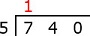Step 2:

Multiply 5 × 1 = 5. Subtract 7 – 5.Step 3:

Bring down 4.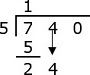Step 4:

Divide 24 ÷ 5 = 4, Remainder = 4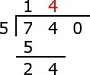Step 5:

Multiply 5 × 4 = 20. Subtract 24 – 20 = 4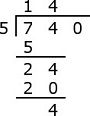Step 6:

Bring down 0.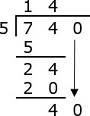Step 7:

Divide 40 ÷ 5 = 8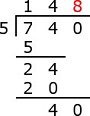Step 8:

Multiply 5 × 8 = 40. Subtract 40 − 40 = 0